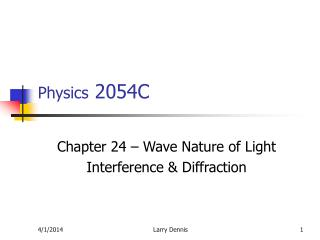Download PresentationPhysics 2054C# Physics 2054C - PowerPoint PPT Presentation

Download Presentation##### Physics 2054C

Download Policy: Content on the Website is provided to you AS IS for your information and personal use and may not be sold / licensed / shared on other websites without getting consent from its author. While downloading, if for some reason you are not able to download a presentation, the publisher may have deleted the file from their server.

- - - - - - - - - - - - - - - - - - - - - - - - - - - E N D - - - - - - - - - - - - - - - - - - - - - - - - - - -
##### Presentation Transcript

1. Physics 2054C Chapter 24 – Wave Nature of Light Interference & Diffraction Larry Dennis

2. Sources Interference - Causes • Two waves traveling slightly different distances to get to the same point • Light shining on one or more openings • Light reflected from two surfaces Larry Dennis

3. Constructive Interference Light Source Viewing Screen D Condition for maximum light intensity isDsin = n, n =0, 1, 2, … Larry Dennis

4. Destructive Interference Viewing Screen Light Source D Condition for minimum light intensity is: Dsin = (n+½), n =0, 1, 2, … Larry Dennis

5. n = 0 n = 1 Interference Pattern Maxima: Dsin = n Minima: Dsin = (n+½ ) Larry Dennis

6. Diffraction Properties • Pattern produced by a single source due to its finite size. • Important when the source size is comparable to the wavelength. • Minima: dsin = m, m = 1,2,3 … Larry Dennis

7. d sin = - d sin =  Diffraction Modulates Interference Pattern Larry Dennis

8. Diffraction + Interference Larry Dennis

9. Thin Film • Phase changes due to different distances traveled. • Phase changes due to reflections • When light moving in a medium of low index of refraction is reflected from a medium of higher index of refraction the reflected waves undergoes a phase change of 180°. Larry Dennis

10. 1 2 glass, n = 1.44 glass, n = 1.44 Example • How thick (minimum) should the air layer be between two flat glass surfaces if the glass is to appear bright when 480 nm light is incident normally? • How thick (minimum) should the air layer be between these two glass surfaces if the glass is to appear dark? Larry Dennis

11. 1 2 glass, n = 1.44 glass, n = 1.44 Example • Phase difference between rays 1 and 2 comes from two sources: • Phase change of  in ray 2 at the bottom air/glass surface. • Additional distance traveled by ray 2.  = 2*2nt/ • Net phase difference between ray’s 1 and 2 is: •  = 2*2nt/ +  • This results in constructive interference when it is an integer multiple of 2 ( or 2m ). • This results in destructive interference when it is an odd integer multiple of  ( or 2(m+½) ). Larry Dennis

12. 1 2 glass, n = 1.44 glass, n = 1.44 Example • We can equate the net phase difference between ray’s 1 and 2 and the conditions for constructive and destructive interference • For constructive interference: •  = 2*2nt/ +  = 2m  2nt/ + ½ = m • or 2nt/ = m - ½ (NOTE: t must be positive, so m>0) • For destructive interference: •  = 2*2nt/ +  = 2(m+½)  2t/ + ½ = (m+½) • or 2nt/ = m Larry Dennis

13. CAPA 7: Interferometer D As the volume fills with gas the wavelength of the light is reduced from  to /n. In the process an additional N = 200 waves are squeezed into the volume. The number of waves already in the volume is 2D/ (the volume has an effective length of 2D since the light goes through twice). So: The change in the number of waves in the volume is: N = 2Dn/ - 2D/  N = 2D(n-1)  n-1 = N/2D Note that this differs from the formula discussed in class. Larry Dennis

14. Polarization • Light is an electromagnetic wave. • Electric field determines the direction of lights polarization. • Normally, the direction of the electric field points randomly in all directions. • For polarized light, the electric field vector of all the photons point in the same direction. Larry Dennis

15. Polarizers • Materials that transmit light polarized in a particular direction (along the axis of polarization). • Transmitted light Iout = Iincos2(). Transmission Axis Can be in any direction. Outgoing light is reduced intensity and polarized along the transmission axis. Larry Dennis

16. Example: Two polarizers • Unpolarized light goes into 1st Polarizer: • Polarized light comes out  along transmission axis. • Intensity is ½ of what went in. • Polarized light goes into second polarizer: • Polarized light comes out  along transmission axis of 2nd polarizer. • Intensity reduced by an additional factor of cos2() of the angle between the two transmission axes. Larry Dennis

17. Example: Two polarizers • Iout = ½ Iincos2() • 0° ½ intensity • 90°  no light out Larry Dennis